Article

# Analysis of the Influence of Terrain Orientation on the Design of PV Facilities with Single-Axis Trackers

1
Department of Mechanics, University of Córdoba, 14071 Cordoba, Spain
2
Department of Electronic and Computer Engineering, Campus of Rabanales, University of Córdoba, 14071 Cordoba, Spain
3
Physics for Renewable Energies Research Group, University of Córdoba, 14071 Cordoba, Spain
4
Department of Applied Physics, Radiology and Physical Medicine, University of Córdoba, 14071 Cordoba, Spain
5
Department of Electrical Engineering and Automatics, University of Córdoba, 14071 Cordoba, Spain
*
Author to whom correspondence should be addressed.
Appl. Sci. 2020, 10(23), 8531; https://doi.org/10.3390/app10238531
Received: 5 November 2020 / Revised: 23 November 2020 / Accepted: 27 November 2020 / Published: 29 November 2020

## Abstract

This paper investigates how to optimally orient the photovoltaic solar trackers of an axis parallel to the terrain, applying the sky model of Hay–Davies. This problem has been widely studied. However, the number of studies that consider the orientation (inclination and azimuth of the terrain) is very limited. This paper provides an examination of incident solar irradiance that can be extended to terrain with variable orientation and in consideration of different azimuths of the axis of rotation. Furthermore, a case study of the south of Spain is provided, considering different inclination and orientation terrain values. The results obtained in this study indicate, as a novelty, that for lands that are not south facing, the rotation axis azimuth of solar trackers should be different from zero and adjusted to the same direction as the land azimuth in order to maximize energy production. Annual energy production is sensitive to changes in the rotation axis azimuths of solar trackers (an influence of around 3% of annual energy production).

## 1. Introduction

At present, population growth, deficiencies in natural resources and global warming are producing challenges around the world. In recent years, various initiatives have been working on the development or replacement of the current power grid to obtain greater efficiency in the electrical system and a reduction in energy waste by reducing losses during distribution . Such a system would contribute to the promotion of renewable resources and the minimisation of the environmental impact in the future. Therefore, there is a global requirement to preserve the environment and to improve the penetration of alternative energy resources . Within the framework of renewable energies, solar energy is the form of energy that has shown the most remarkable growth in recent years thanks to the reduction of costs and to legal requirements in many countries [3,4]. Similarly, photovoltaic (PV) technology is one of the current technologies with a better future projection due to its simplicity and scalability and the continuous manufacture, operation and maintenance reduction costs it enables for solar panels .
However, the lack of the linearity in the solar energy received by solar panels, mainly caused by Earth–Sun relative displacement, is a disadvantage to consider. It is necessary to redefine solar tracking systems by increasing the solar irradiance capture enabled by PV collectors and therefore improve energy production .
Classified according to the type of movement, the following systems can be considered: single-axis tracking systems, in which a mobile element adapts its position by rotating around a fixed axis; and two-axis tracking systems, in which the collector plane rotates around two fixed axes, allowing an orientation towards any direction of the celestial sphere [7,8].
Several studies have analysed the efficiency of energy production using two-axis trackers compared with single-axis tracking and fixed panels [9,10,11]. Bahrami et al.  determined that the increase in solar production of a PV plant with two-axis trackers compared to a system with single-axis trackers at the same latitude is 0.42–23.4%. Similarly, the improvement compared to a fixed-panel system is around 17.22–31.23%. Other authors, such as Hua et al. , concluded that fluctuations in energy production can be reduced depending on the tracker distribution in the PV plant.
With regard to movement strategies, two types can be distinguished: those based on information from pyranometers and those based on mathematical statements, determined by terrestrial and solar movement. For this latter option two approaches can be considered. For the first, the sun’s position is predicted by spherical trigonometry [12,13,14], and for the second, it is acquired by vectorial calculation [15,16]. This article uses vectorial calculation.
The most frequently followed strategy in solar tracking is that based on the astronomical model. This method aims to minimise the angle composed by direct sunrays and the normal vector to the collector plane, thereby increasing the direct portion of solar irradiance. However, this strategy is not optimal for the PV energy case since it does not involve the remaining portions (diffuse and reflected) which also contribute to energy production. This issue was thoroughly studied by Duffie and Beckman , who found that on cloudy days astronomical tracking obtained an irradiance lower than fixed panels because during those days direct radiation fails.
PV plant yields can be highly affected when panels are shaded by each other [17,18,19]. Two consequences can be derived from the shading effect on the panels. The first consequence is the reduction of captured irradiance since the direct component does not reach the cell surface. The second consequence is the increase in temperature of the shaded cell since it works as a resistive load absorbing heat from the adjacent cells which thus accelerates its deterioration [18,20]. In this respect, it would be advisable to arrange the location of the panels in such a way that there is no intershading. However, given the high price of the terrain and the increasingly low price of the panels, the most common solution is to construct PV plants with suitable distances between the panels that produce shade . This important scientific challenge has been widely studied by many authors who are interested in the reduction in PV production due to shade from the panels [17,18,21,22].
In addition, solar geometry and PV plant design also affect energy production. Solutions which aim at simulating all the relevant aspects of this issue therefore demand significant calculation times [23,24]. However, authors such as Saint-Drenan and Barbier  have started to work on the optimisation of this issue using a model with a low computational load. This model can maintain the required accuracy levels using a few input parameters. However, it has only been tested in two PV plants. The authors therefore recommend extending the validation of this model to other facilities and geometries. Consequently, it is necessary to continue studying the influence of shading on energy production.
Under this paradigm, the backtracking method can provide adequate solutions for the intershading problem in PV plants , especially during sunrise and sunset when solar beams are very low and there is greater intershading between PV modules. This method varies the optimum angle of greatest solar incidence to prevent the back panels from being shaded (Figure 1). Although the result of using backtracking is a lower angle of incidence of solar collection, it is more favourable than the projection of shadows among adjacent solar panels .
Backtracking has become a technique used to increase energy production in photovoltaic plants in cloudy periods. The diffuse component of solar radiation is much more relevant in these cases and “approaches” that ignore this component (such as astronomical ones) are thus no longer optimal.
This is not the only advantage of employing backtracking. There is also the resulting reduction in hot spots on the trackers, which increases their life span and reduces breakdowns. Backtracking also improves the ground cover ratio (GCR) of the installations, which has the effect of reducing the economic impact with regard to the soil required when building plants .
For these reasons, there is a niche in scientific work focused on the development of options utilising backtracking and on the optimisation of solutions responding to the challenges posed by solar panels which are not exclusively focused on classical tracking [9,26,28,29].
From this perspective, work based on tracking has been undertaken with the aim of optimising production throughout the movement of a panel path, including the backtracking periods . To achieve this, the search is underway for a panel path that maintains the optimum production under the premise that the panel is isolated and therefore does not receive shade from any adjacent panel. Once this objective has been considered, a condition can be established to verify the possible shading between photovoltaic panels. After these two steps, the shaded orientations can be indicated against those that are not shaded. Thus, a panel path that optimises production, including the non-shading of modules, can be established.
Since it is difficult to find a completely horizontal location for photovoltaic plants, further study and deeper knowledge of photovoltaic trackers are required to offer optimisation guidelines for solar capture. The present study was undertaken to include: (a) mathematical modelling of solar capture on trackers; (b) programming that allows automation of the calculations; (c) simulations of case studies; and (d) analysis of optimal conclusions. In light of this, conclusions were drawn on orientations, land inclinations and distances between modules, etc., in terms of produced energy.
Figure 2a,b represent angles χ (azimuth) and β (inclination) for a generic terrain. The angle γ (azimuth of the collector rotation axis) is also represented. This study, based on the Hay–Davies model, characterises solar radiation on trackers using angles χ, β and γ.
The remainder of this paper is organised as follows. In Section 2 the model used, the mathematical optimisation bases, the backtracking method and the relevant software development are presented. Section 3 outlines how the methodology was tested in Córdoba, Spain, and the results are discussed. In Section 4, conclusions are drawn based on the restrictions shown and potential further research is indicated.

## 2. Materials and Methods

#### 2.1. Astronomical Bases and Irradiance Model

Knowing the concrete position of the sun at each moment of the day and on each day of the year is fundamental for determining the direction of solar beams. It is possible to determine the position of the sun accurately by using astronomical geometry. For this purpose, a solar vector was defined, expressed with respect to a terrestrial reference system (Figure 2) and formed by the axes Ox, towards the west; Oy, towards the south; and Oz in the zenithal direction. The position was determined by Equations (1) and (2):
$s → = s x i → + s y j → + s z k →$
$s → = s i n Ω t · c o s δ i → + ( c o s Ω t · c o s δ · s i n φ − s i n δ · c o s φ ) j → + ( c o s Ω t · c o s δ · c o s φ + s i n δ · s i n φ ) k →$
where $δ$ represents the solar declination, $φ$ the latitude and $Ω t$ the hour angle. This angle was calculated as the Earth’s rotation speed, 2π/24 rad/h, for the hours since solar noon .
In PV technology, all irradiance components (direct, diffuse and reflected irradiance) are usable. Traditionally, the astronomical model has been used to determine the position of the sun. Applying the astronomical model to solar tracking means that the angle formed between the direct solar rays and the normal angle to the collector’s surface $θ$ must be as low as possible. With astronomical tracking, the value of the direct irradiance component is maximised, which is appropriate for applications focused on this component (such as concentration technologies). Therefore, this type of tracking is not the most suitable for the purposes of this study. For instance, on cloudy days, when the solar disk is not visible and direct radiation does not reach the collectors, those located on a fixed horizontal position collect more energy than those using astronomical tracking. Therefore, to study the influence of diffuse and reflected components on solar tracking, several sky models have been proposed.
The Hay–Davies method  was used for the calculations in this work because it adequately describes the anisotropy of radiation  in addition to being notable for its simplicity, as opposed to other more complex models such as those of Muneer  and Perez , and for obtaining high quality results [35,36,37]. This model establishes that a determined fraction of diffuse irradiance is directed according to the direction of the solar disk. Models such as that of Hay–Davies  describe the irradiance affecting a solar panel by considering its three components, direct, diffuse and reflected irradiance, but take into account the fact that the diffuse component has a preferential direction in which the diffuse radiation is greater. According to Hay , the mathematical expression of the model is given by Equation (3), where the first term indicates the direct irradiance IB, the second term refers to the diffuse irradiance ID and the third term corresponds to the reflected component, taking into account the visible soil fraction and the albedo, $ρ$. IB and ID are determined by the Collares–Pereira model .
$I = c o s θ c o s θ z I B + [ ( c o s θ c o s θ z ) I B I O H + ( 1 − I B I O H ) 1 + c o s ξ 2 ] I D + ρ 1 − c o s ξ 2 ( I B + I D )$
where $ξ$ is the inclination angle of the collector and $θ Z$ is the solar zenith angle, that is, the angle between the solar vector and the zenith axis.

#### 2.2. Optimisation of Collector Orientation

The aim of this study was to establish guidelines for designing facilities with solar trackers on a rotation axis $e →$. This axis was considered as parallel to the terrain; no restrictions were initially imposed on it. The general results are valid for any disposition.
In this study there were two degrees of freedom for the orientation of a surface, the azimuth $χ$ and inclination $β$. In the case of single-axis trackers, there would only be the elevation. The orientation of a panel was characterised by a single vector $n →$, normal to the surface of the panel. The objective was therefore the calculation of $n →$ such that that the solar capture was the maximum.
Expanding on the treatment of the vectors, the cosines of the angles $θ$, $θ Z$ and $ξ$ that appear in the expressions of the different models of the irradiance are expressed as the scalar products of $s →$, and $k →$:
Substituting the previous expressions into the Hay–Davies model  gives the following:
$I = s → · n → s → · k → I B + [ ( s → · n → s → · k → ) I B I O H + ( 1 − I B I O H ) 1 + k → · n → 2 ] I D + ρ 1 − k → · n → 2 ( I B + I D )$
To optimise the irradiance , the Lagrange multiplier method was used with the following restrictions defined: , $e →$ being the ground normal vector.
The differential is described by:
$d Φ = [ ∂ I ∂ ( s → · n → ) s → + ∂ I ∂ ( k → · n → ) k → − 2 λ n → − μ e → ] · d n → + [ 1 − n → · n → ] d λ + d μ [ 0 − e → · n → ] + [ 1 − e → · e → ] d ν$
by matching the brackets to zero.
$d Φ = [ ∂ I ∂ ( s → · n → ) s → + ∂ I ∂ ( k → · n → ) k → − 2 λ n → − μ e → ] · d n → + [ 1 − n → · n → ] d λ + d μ [ 0 − e → · n → ] + [ 1 − e → · e → ] d ν$
By naming
$u → = ∂ I ∂ ( s → · n → ) s → + ∂ I ∂ ( k → · n → ) k →$
three vectors $u → , n →$ and $e →$ appear. These vectors fulfil the relation:
$u → − 2 λ n → − μ e → = 0$
So, they are linearly dependent and therefore coplanar.
As $n →$ is perpendicular to $e →$, it can be expressed more easily.
$n → = u → − ( u → · e → ) · e → | u → − ( u → · e → ) · e → |$
Figure 3 represents the plane formed by the vectors $u →$, $n →$ and $e →$.
Replacing the vectors $e →$ and $u →$ with their expressions results in the equation that defines the vector $n →$:
This result makes it possible to calculate the optimal direction of the normal vector for any irradiance model in any direction of the rotation axis and for different positions of the sun. By replacing the values of the partial derivatives in Equation (14) with those obtained from Equation (7), we get:
Utilising Equation (16), the values of $n →$ can be obtained at any given time for the optimum irradiance incidence.
For example, for the simplest case of a direct irradiance model which only takes into account the first term of Equation (3), and with the rotation axis of the solar panels assumed to be horizontal to the ground, a normal vector like the following results, where the vector $e →$ is:
$β$ being the inclination of the terrain, $χ$ the azimuth of the terrain and $γ$ the azimuth of the rotation axis collector.

#### 2.3. Backtracking

In the previous section, the methodology used to determine the orientation of the solar tracker in order to obtain the maximum solar capture was detailed. This section describes the method used to prevent a solar tracker from shading an adjacent tracker. Only two factors needed to be considered for this method: the position of the sun and the orientation of the panels. To study intershading it is convenient to use an auxiliary reference system where the representation plane is perpendicular to the tracker axis. For this purpose, the vector $q →$ was defined as:
where $q →$ is a vector perpendicular to the ground normal vector $e →$, as well as to the rotation axis vector $n T →$. The solar vector $s →$ and the plane normal vector in the optimal position $n →$ were named $s ′ →$ and $n ′ →$ in the reference system composed by (Figure 4).
The procedure used to estimate whether a solar panel B would shade an adjacent panel A was to project the shadow of panel B onto the straight line on which the adjacent panel A lay (Figure 5). Since the sun’s rays are parallel to each other, the projection of one point was sufficient. First, a vector was defined from the centre of solar panel B in the direction of the solar rays $τ s ′ →$. Next, the central point of solar panel A was projected onto the centre of panel B, defining $a →$. The examination of this vector enables the determination of whether shading will occur.
In order to obtain the vector $a →$, both terms were multiplied by the vector $n ′ →$:
Substituting the value of $τ$ from Equation (19) into Equation (18) results in:
$d → = a → + n ′ x · d s ′ x · n ′ x + s ′ z · n ′ z s ′ →$
Vector $a →$ can be obtained according to the following expression:
$a → = ( d − n ′ x · d s ′ x · n ′ x + s ′ z · n ′ z · s ′ x ) · p → − n ′ x · d s ′ x · n ′ x + s ′ z · n ′ z · s ′ z · n T →$
As mentioned above, the examination of vector $a →$ enables the determination of whether shading will occur. If the module of the vector is less than the width of the panel, shading will occur.
$| a → | < h$
To avoid this situation, backtracking was used to look for a new orientation of the panels that prevented shading. The new orientation was intended to involve a minimum deviation from the optimal capture orientation. The new inclination angle $α$ was that which caused the shading of a panel to fall on the edge of the adjacent panel (Figure 6).
This inclination angle $α$ was calculated based on a triangle formed by the line that joins the center of the two panels $d$, the projection of the centre of the panel on the line of the adjacent $τ s ′ →$ and the distance that corresponds to the module of vector $a →$ in Figure 5, which in Figure 6 is equivalent to the width of the panels,.
The angle $ζ$ is the one between the solar vector $s ′ →$ and the $Χ$ axis:
$γ = arctan s ′ z s ′ x$
The value of the angle $δ$ can already be calculated as:
$s i n δ = s i n ζ · d a$
Therefore, the inclination angle of the solar panel can be calculated as follows:
The normal vector that leads to the determination of the orientation of the panel in order to avoid shading is:

#### 2.4. Software Applications for Analysis

To calculate the irradiance received by each solar panel, a function was implemented in Visual Basic for Applications (VBA) for Excel in which the calculations described above were developed. As seen in Figure 7, the function was based on several input parameters (latitude, Julian day, geometrical values, irradiance model considered) and provided an irradiance value every three minutes. Some restrictions, such as whether it as daytime, the maximum radiation related to the used model and eventual shading with backtracking response were assumed.

## 3. Results and Discussion

The practical results provided the value of the estimated annual radiation using the Hay–Davies model  for various land configurations. All the calculations were made for the province of Córdoba, Spain, therefore assuming the same geographical values (latitude = 37.75492° N; longitude = 5.04548° W) and the same climatic values throughout the year, varying only the values of inclination $β$ and azimuth $χ$ for a fixed panel width and separation between panel lines .
For each inclination $β$ (from 0° to 45°, 1° increment) and terrain azimuth (from −60° to 60°, 5° increment), the rotation axis was oriented with a wide range of azimuth γ (from −20° to 20°, 2° increment).
Terrains with negative inclinations were not considered since PV plants are not commonly installed in shaded areas.
For facilities located on terrain with a null azimuth (oriented south), independently of its inclination even if it is null, the optimal orientation of the axis of rotation is southward (γ = 0°).
For each value of the inclination of the terrain $β$, it was also observed that the maximum value of irradiance was obtained when the azimuth of the terrain $χ$ was 0°. That is, the optimal situation is when the terrain is oriented south and the axis of rotation is directed in the same direction. If the terrain has another orientation, the value of the optimal angle towards which the axis of rotation should be oriented would move in that same direction. This fact is more evident at higher values of the terrain inclination $β$, although in no case will the azimuth axis γ be as high as that of the terrain $χ$.
The results for a case study with a $β$ = 15° inclination are presented in Figure 8. Figure 8a shows the variation of radiation with regard to γ when the azimuth of the terrain $χ = 0 °$. However, when the terrain was not oriented to the south, as shown in Figure 8b in which the terrain was turned = 30°, the maximum radiation was obtained by orienting the axis with an azimuth γ of 6° towards the south. The losses with regard to the maximum radiation obtained in a terrain are shown in Figure 8c, where it is again verified that, by orienting the axis at 6°, these losses can be minimised. Finally, Figure 8d shows the optimal orientation to direct the axis for the different values of the terrain azimuth $χ$. In this way it is possible to estimate the orientation that achieves optimal radiation considering the azimuth of the terrain $χ$.
As already mentioned, for each inclination value the maximum irradiance value was obtained when the terrain had an angle $χ = 0 °$. In addition, as the inclination of the terrain increased, the radiation affecting the panels was greater, as seen in Figure 9. The explanation can be found in the latitude for which the calculations were made and in the fact that the direction of the sun is not perpendicular to the horizontal plane. The inclination of the terrain influenced the radiation, increasing until a value of $β$ of around 21°, where the maximum was reached.
The difference observed between the radiation value for a particular axis azimuth and the maximum capture obtained in the optimum direction was greater as the inclination of the terrain increased. Thus, in low-inclination terrains the difference was low and while for steeper inclinations the losses were considerable. Figure 10 shows that, in the specific case of $β$ = 20°, the losses caused by a bad choice of rotation axis direction can reach values above 7%.
Figure 11 shows the losses for the conditions γ = 0° and $β$ = 20°, considering an azimuth range $χ$ from −60° to 60°. For terrains that are also oriented towards the south, or close to it, the losses will be zero or very low, but as the azimuth of the terrain increases, the losses become considerable.
Thus, it is possible to establish a relationship between the sensitivity of the collector axis azimuth γ and the inclination $β$ and azimuth of the terrain $χ$. It is worth highlighting the novelty of this result since no references have been found in the literature suggesting this relationship between variables.
Another key factor when configuring the layout of the collectors was the separation between them. This fact had an influence since the greater the panel separation is, the fewer shading effects are produced and the fewer losses due to a decrease in production. On the other hand, it was not possible to separate the rows of collectors as much as desired, since the costs associated with a higher terrain occupation increase rapidly . Figure 12 shows that, depending on this parameter, the irradiance demonstrated asymptotic behaviour. This was because the shadow effects of one collector on another, when separated by a sufficient distance, were no longer perceived, thus resulting in an irradiance value like that of an isolated collector without adjacent collectors.

## 4. Conclusions

In this paper, the solar capture on horizontal one-axis trackers was mathematically modelled, thus establishing the rotation axis orientation for any irradiance model. Backtracking was used to avoid the shading of some panels on adjacent ones, modifying their orientation. The results obtained, combined with those in the literature, amount to an advance in the knowledge on photovoltaic trackers with axes parallel to the terrain, allowing an optimal design for terrains without the horizontality described in previous works.
A program and a subroutine were implemented in VBA to automatically calculate the radiation affecting a solar tracker for different terrain configurations and for which it was possible to vary the characteristics of the installation, namely the rotation axis orientation and the ratio dimension of the panels $h$ and the separation $d$ between adjacent rows. Simultaneously, the program and subroutine provided the optimal azimuth γ value for the rotation axis to be suitably directed and the loss values for each possible maximum radiation value for each inclination $β$ of the terrain.
The calculations of the amounts of radiation affecting solar panels, assuming fixed width and distance between lines of panels and varying terrain inclinations $β$ and azimuth $χ$, were made in Córdoba, Spain.
For any given value regarding the terrain inclination $β$, it was verified that a higher value of radiation was obtained for terrain that was oriented south ($χ = 0 ° )$. The optimum direction for the rotation axis collector γ should also be southwards.
An important contribution of this research is the relationship established between the azimuth γ of the collector axis and the inclination $β$ and azimuth of the terrain. For terrain that is not south facing, the rotation axis azimuth γ of the solar trackers should be different from zero and varies in the same direction as the land azimuth $χ$. If the axis of rotation is positioned in a different direction to the optimum, losses in potential energy production arise. These losses vary according to the terrain inclination $β$. The greater the difference between the optimum azimuth γ and the terrain inclination $β$, the greater the production losses will be.
Another important conclusion drawn from this research concerns the collector layout on the power plant surface. As the distance $d$ between rows of collectors increases, a greater amount of radiation is generated. Such growth is asymptotic.
Overall, some progress has been achieved towards the objective of deepening knowledge concerning photovoltaic trackers. Consequently, guidelines were proposed for solar capture optimisation in photovoltaic plants. This work opens up further lines of research into the geometric layout optimisation of solar trackers involving more complex models, such as those of Perez  and Muneer , and real databases.
As a result of the aforementioned conclusions, it is considered that this study can be the basis for further work, such as the study of one-axis solar trackers located on terrains with irregular topographies or of the collector distribution system, considering potential uses of the land.

## Author Contributions

Conceptualization, F.J.G.-U., I.M.M.-G. and R.L.-L.; methodology, I.M.M.-G. and L.M.F.-A.; software, J.M.J.-M.; validation, I.M.M.-G. and L.M.F.-A.; formal analysis, L.M.F.-A., I.M.M.-G. and R.L.-L.; bibliographic search, F.J.G.-U. and L.M.F.-A.; data curation, J.M.J.-M. and R.L.-L.; writing—original draft preparation, L.M.F.-A., F.J.G.-U. and I.M.M.-G.; writing—review & editing, F.J.G.-U., I.M.M.-G., R.L.-L. and L.M.F.-A.; supervision, I.M.M.-G., R.L.-L. and L.M.F.-A. All authors have read and agreed to the published version of the manuscript.

## Funding

This research received no external funding.

## Acknowledgments

This research was partially technically supported by the CLARA Project (European Union’s Horizon 2020 research and innovation programme under Grant Agreement No 730482). The authors thank Azul y Verde Energía y Sostenibilidad S.L. for their collaboration in this research. The authors also thank Simon Craig for his contribution to the English edition.

## Conflicts of Interest

The authors declare no conflict of interest.

## Abbreviations

 $a →$ projection vector of the solar panel being studied for shading $d →$ distance between the centers of two adjacent solar collectors $e →$ unit vector contained in the collector rotation axis $G C R$ ground cover ratio $h$ collector width $i → , j → , k →$ unit vectors associated with a local Cartesian system $I$ global solar irradiance on the tilted collector $I B$ direct solar irradiance on the horizontal plane diffuse solar irradiance extraterrestrial irradiance $n →$ normal vector to the surface $n ′ →$ optimal normal vector to the surface components of optimal normal vector to the surface $n T →$ normal terrain vector $p →$ unit vector perpendicular to ground normal vector $e →$ $q →$ perpendicular vector to $n T →$ and $e →$ $s →$ solar vector components of solar vector $s ′ →$ optimal solar vector components of optimal solar vector $u →$ irradiance gradient Greek Letters α elevation angle of the collector $β$ inclination angle of the terrain γ azimuth angle of the collector rotation axis $δ$ solar declination $ξ$ inclination angle of the collector $θ$ angle of incidence of sunbeams on the inclined plane $θ z$ solar zenith angle Lagrange multipliers $ρ$ albedo $τ$ scalar multiplying solar vector to accomplish parallelogram rule $φ$ latitude $Φ$ Lagrange function $χ$ azimuth of the terrain $Ω$ Earth’s rotation speed

## References

1. Carballo, J.A.; Bonilla, J.; Roca, L.; Berenguel, M. New low-cost solar tracking system based on open source hardware for educational purposes. Sol. Energy 2018, 174, 826–836. [Google Scholar] [CrossRef]
2. United Nations. The Sustainable Development Goals Report 2019; United Nations Publications (Department of Economic and Social Affairs): Herndon, VA, USA, 2019. [Google Scholar]
3. Ribó-Pérez, D.; Van der Weijde, A.H.; Álvarez-Bel, C. Effects of self-generation in imperfectly competitive electricity markets: The case of Spain. Energy Policy 2019, 133, 110920. [Google Scholar] [CrossRef]
4. International Renewable Energy Agency. Renewable Power Generation Costs in 2019; International Renewable Energy Agency: Abu Dhabi, UAE, 2020; ISBN 978-92-9260-244-4. [Google Scholar]
5. Kavlak, G.; McNerney, J.; Trancik, J.E. Evaluating the causes of cost reduction in photovoltaic modules. Energy Policy 2018, 123, 700–710. [Google Scholar] [CrossRef]
6. Hua, Z.; Ma, C.; Lian, J.; Pang, X.; Yang, W. Optimal capacity allocation of multiple solar trackers and storage capacity for utility-scale photovoltaic plants considering output characteristics and complementary demand. Appl. Energy 2019, 238, 721–733. [Google Scholar] [CrossRef]
7. Hafez, A.Z.; Yousef, A.M.; Harag, N.M. Solar tracking systems: Technologies and trackers drive types—A review. Renew. Sustain. Energy Rev. 2018, 91, 754–782. [Google Scholar] [CrossRef]
8. Nsengiyumva, W.; Chen, S.G.; Hu, L.; Chen, X. Recent advancements and challenges in Solar Tracking Systems (STS): A review. Renew. Sustain. Energy Rev. 2018, 81, 250–279. [Google Scholar] [CrossRef]
9. Koussa, M.; Cheknane, A.; Hadji, S.; Haddadi, M.; Noureddine, S. Measured and modelled improvement in solar energy yield from flat plate photovoltaic systems utilizing different tracking systems and under a range of environmental conditions. Appl. Energy 2011, 88, 1756–1771. [Google Scholar] [CrossRef]
10. Bahrami, A.; Okoye, C.O.; Atikol, U. The effect of latitude on the performance of different solar trackers in Europe and Africa. Appl. Energy 2016, 177, 896–906. [Google Scholar] [CrossRef]
11. Abdallah, S.; Nijmeh, S. Two axes sun tracking system with PLC control. Energy Convers. Manag. 2004, 45, 1931–1939. [Google Scholar] [CrossRef]
12. Braun, J.E.; Mitchell, J.C. Solar geometry for fixed and tracking surfaces. Sol. Energy 1983, 31, 439–444. [Google Scholar] [CrossRef]
13. Duffie, J.A.; Beckman, W.A. Solar Engineering of Thermal Processes; John Wiley & Sons, Inc.: Hoboken, NJ, USA, 2013; ISBN 9781118671603. [Google Scholar]
14. Narvarte, L.; Lorenzo, E. Tracking and ground cover ratio. Prog. Photovolt. Res. Appl. 2008, 16, 703–714. [Google Scholar] [CrossRef]
15. Parkin, R.E. Solar angles revisited using a general vector approach. Sol. Energy 2010, 84, 912–916. [Google Scholar] [CrossRef]
16. Sproul, A.B. Derivation of the solar geometric relationships using vector analysis. Renew. Energy 2007, 32, 1187–1205. [Google Scholar] [CrossRef]
17. Fan, X.; Deng, F.; Chen, J. Voltage band analysis for maximum power point tracking of stand-alone PV systems. Sol. Energy 2017, 144, 221–231. [Google Scholar] [CrossRef]
18. Satpathy, P.R.; Sharma, R. Diffusion charge compensation strategy for power balancing in capacitor-less photovoltaic modules during partial shading. Appl. Energy 2019, 255. [Google Scholar] [CrossRef]
19. Seyedmahmoudian, M.; Horan, B.; Soon, T.K.; Rahmani, R.; Than Oo, A.M.; Mekhilef, S.; Stojcevski, A. State of the art artificial intelligence-based MPPT techniques for mitigating partial shading effects on PV systems—A review. Renew. Sustain. Energy Rev. 2016, 64, 435–455. [Google Scholar] [CrossRef]
20. Belhachat, F.; Larbes, C. Modeling, analysis and comparison of solar photovoltaic array configurations under partial shading conditions. Sol. Energy 2015, 120, 399–418. [Google Scholar] [CrossRef]
21. Saint-Drenan, Y.M.; Barbier, T. Data-analysis and modelling of the effect of inter-row shading on the power production of photovoltaic plants. Sol. Energy 2019, 184, 127–147. [Google Scholar] [CrossRef]
22. Perpiñán, O. Cost of energy and mutual shadows in a two-axis tracking PV system. Renew. Energy 2012, 43, 331–342. [Google Scholar] [CrossRef]
23. Deline, C.; Dobos, A.; Janzou, S.; Meydbray, J.; Donovan, M. A simplified model of uniform shading in large photovoltaic arrays. Sol. Energy 2013, 96, 274–282. [Google Scholar] [CrossRef]
24. Martínez-Moreno, F.; Muñoz, J.; Lorenzo, E. Experimental model to estimate shading losses on PV arrays. Sol. Energy Mater. Sol. Cells 2010, 94, 2298–2303. [Google Scholar] [CrossRef]
25. Panico, D.; Garvison, P.; Wenger, H.; Shugar, D. Backtracking: A novel strategy for tracking PV systems. In Proceedings of the Conference Record of the Twenty-Second IEEE Photovoltaic Specialists Conference, Las Vegas, NV, USA, 7–11 October 1991; Volume 1, pp. 668–673. [Google Scholar] [CrossRef]
26. Antonanzas, J.; Urraca, R.; Martinez-de-Pison, F.J.; Antonanzas, F. Optimal solar tracking strategy to increase irradiance in the plane of array under cloudy conditions: A study across Europe. Sol. Energy 2018, 163, 122–130. [Google Scholar] [CrossRef]
27. Fernández-Ahumada, L.M.; Ramírez-Faz, J.; López-Luque, R.; Varo-Martínez, M.; Moreno-García, I.M.; Casares de la Torre, F. A novel backtracking approach for two-axis solar PV tracking plants. Renew. Energy 2020, 145, 1214–1221. [Google Scholar] [CrossRef]
28. Kelly, N.A.; Gibson, T.L. Improved photovoltaic energy output for cloudy conditions with a solar tracking system. Sol. Energy 2009, 83, 2092–2102. [Google Scholar] [CrossRef]
29. Quesada, G.; Guillon, L.; Rousse, D.R.; Mehrtash, M.; Dutil, Y.; Paradis, P.-L. Tracking strategy for photovoltaic solar systems in high latitudes. Energy Convers. Manag. 2015, 103, 147–156. [Google Scholar] [CrossRef]
30. Fernández-Ahumada, L.M.; Casares, F.J.; Ramírez-Faz, J.; López-Luque, R. Mathematical study of the movement of solar tracking systems based on rational models. Sol. Energy 2017, 150, 20–29. [Google Scholar] [CrossRef]
31. Hay, J.E. Calculating solar radiation for inclined surfaces: Practical approaches. Renew. Energy 1993, 3, 373–380. [Google Scholar] [CrossRef]
32. Mousazadeh, H.; Keyhani, A.; Javadi, A.; Mobli, H.; Abrinia, K.; Sharifi, A. A review of principle and sun-tracking methods for maximizing solar systems output. Renew. Sustain. Energy Rev. 2009, 13, 1800–1818. [Google Scholar] [CrossRef]
33. Muneer, T. Solar radiation model for Europe. Build. Serv. Eng. Res. Technol. 1990, 11, 153–163. [Google Scholar] [CrossRef]
34. Perez, R.; Ineichen, P.; Seals, R.; Michalsky, J.; Stewart, R. Modeling daylight availability and irradiance components from direct and global irradiance. Sol. Energy 1990, 44, 271–289. [Google Scholar] [CrossRef]
35. Diez-Mediavilla, M.; De Miguel, A.; Bilbao, J. Measurement and comparison of diffuse solar irradiance models on inclined surfaces in Valladolid (Spain). Energy Convers. Manag. 2005, 46, 2075–2092. [Google Scholar] [CrossRef]
36. Loutzenhiser, P.G.; Manz, H.; Felsmann, C.; Strachan, P.A.; Frank, T.; Maxwell, G.M. Empirical validation of models to compute solar irradiance on inclined surfaces for building energy simulation. Sol. Energy 2007, 81, 254–267. [Google Scholar] [CrossRef]
37. Mubarak, R.; Hofmann, M.; Riechelmann, S.; Seckmeyer, G. Comparison of modelled and measured tilted solar irradiance for photovoltaic applications. Energies 2017, 10, 1688. [Google Scholar] [CrossRef]
38. Collares-Pereira, M.; Rabl, A. The average distribution of solar radiation-correlations between diffuse and hemispherical and between daily and hourly insolation values. Sol. Energy 1979. [Google Scholar] [CrossRef]
Figure 1. Backtracking method.
Figure 1. Backtracking method.Figure 2. Relevant geometry and vectors of an Earth reference system for current study. (a) Perspective view; (b) orthogonal view.
Figure 2. Relevant geometry and vectors of an Earth reference system for current study. (a) Perspective view; (b) orthogonal view.Figure 3. Representation of the plane formed by the vectors $u →$, $n →$ and $e →$.
Figure 3. Representation of the plane formed by the vectors $u →$, $n →$ and $e →$.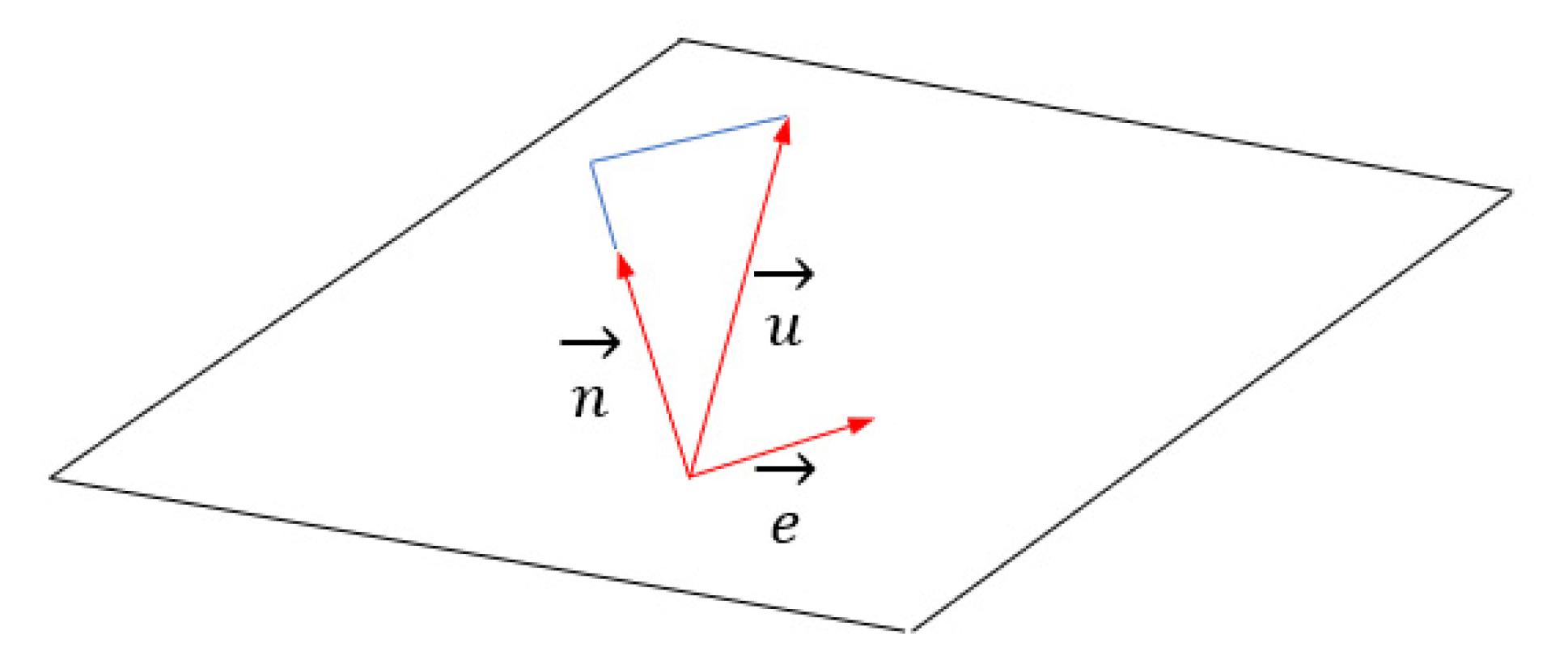Figure 4. Reference system indicating the solar trackers, normal vector and solar vector.
Figure 4. Reference system indicating the solar trackers, normal vector and solar vector.Figure 5. Vectors in the solar trackers reference system.
Figure 5. Vectors in the solar trackers reference system.Figure 6. Angle α avoids the shading of solar panels.
Figure 6. Angle α avoids the shading of solar panels.Figure 7. Flux diagram for Visual Basic for Applications (VBA) function.
Figure 7. Flux diagram for Visual Basic for Applications (VBA) function.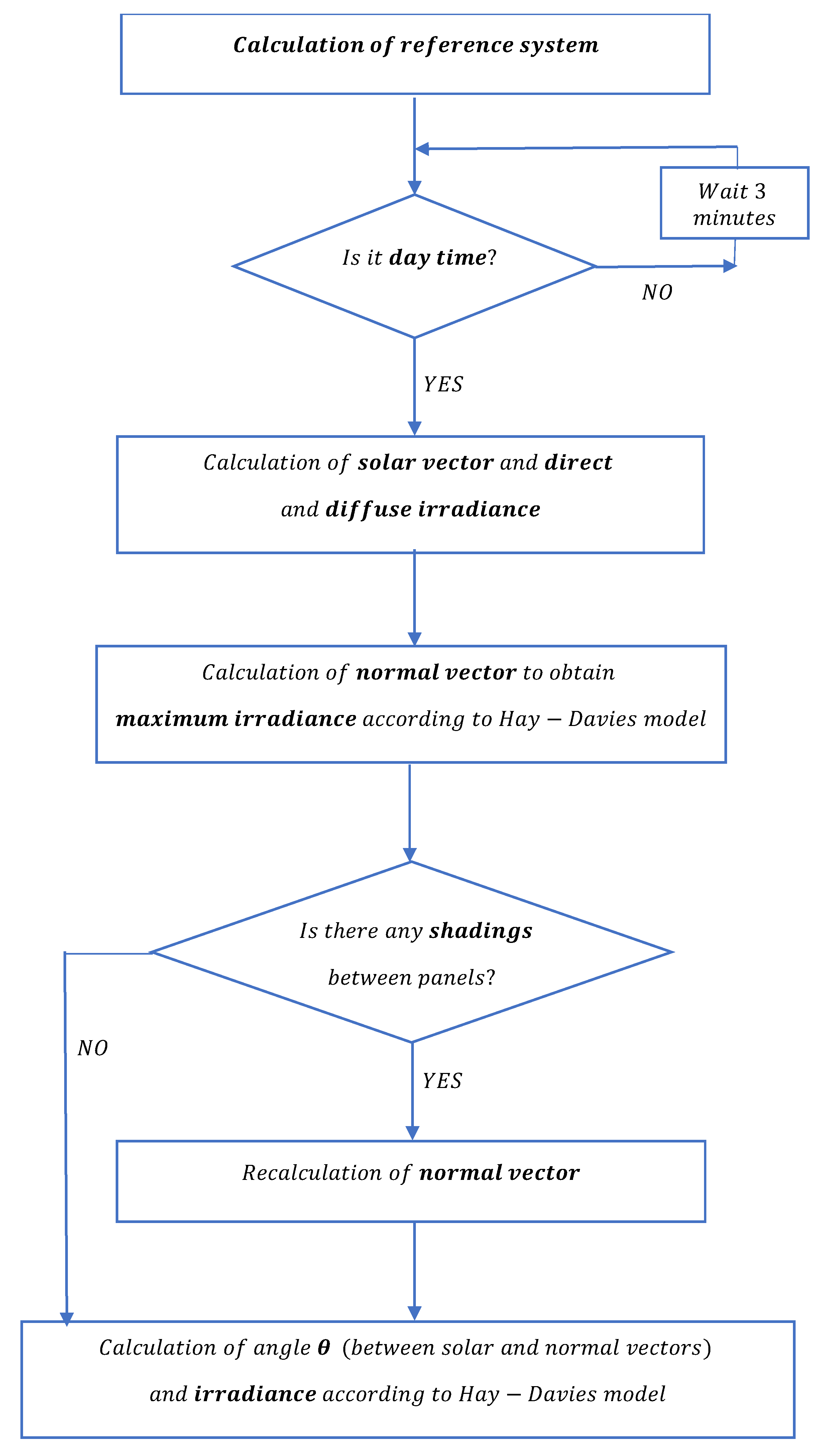Figure 8. Results for a case study for inclination 15°: (a) maximum radiation; (b) radiation variation for 30° azimuth; (c) maximum radiation loss for 30° azimuth; (d) axis azimuth for optimal terrain radiation.
Figure 8. Results for a case study for inclination 15°: (a) maximum radiation; (b) radiation variation for 30° azimuth; (c) maximum radiation loss for 30° azimuth; (d) axis azimuth for optimal terrain radiation.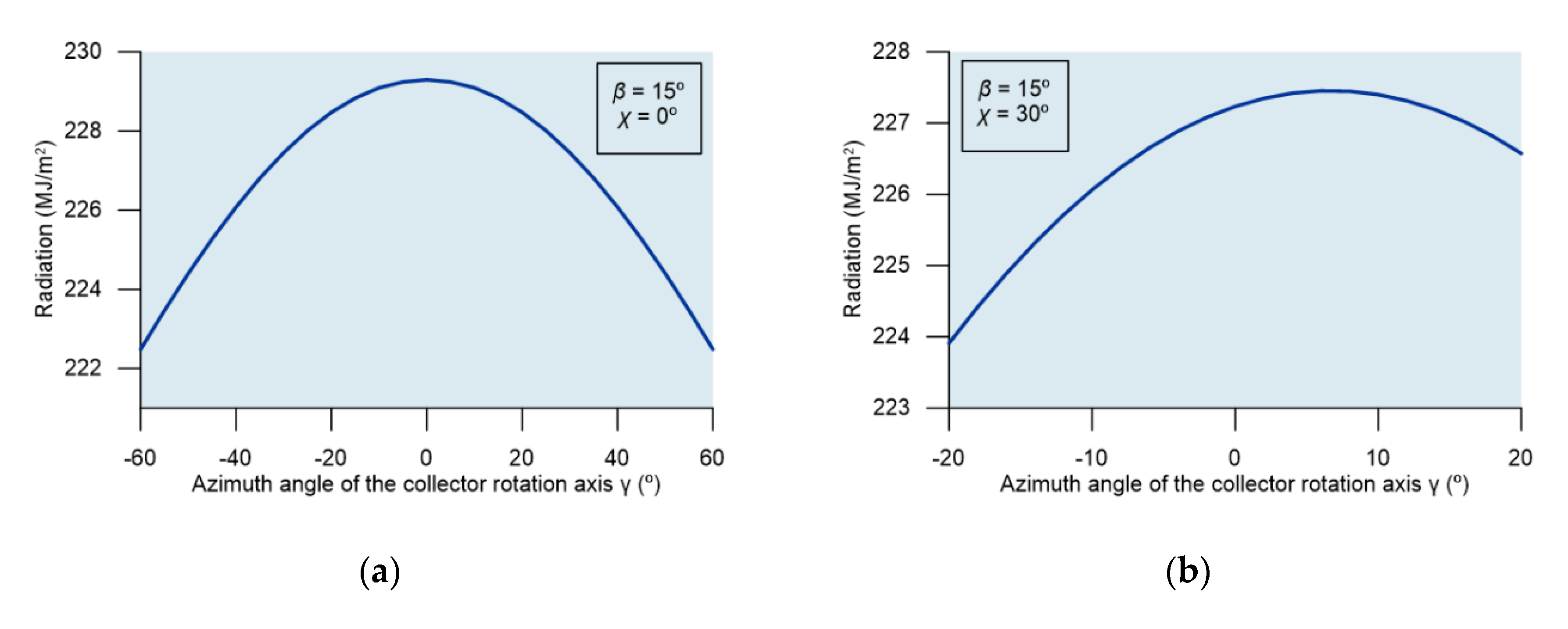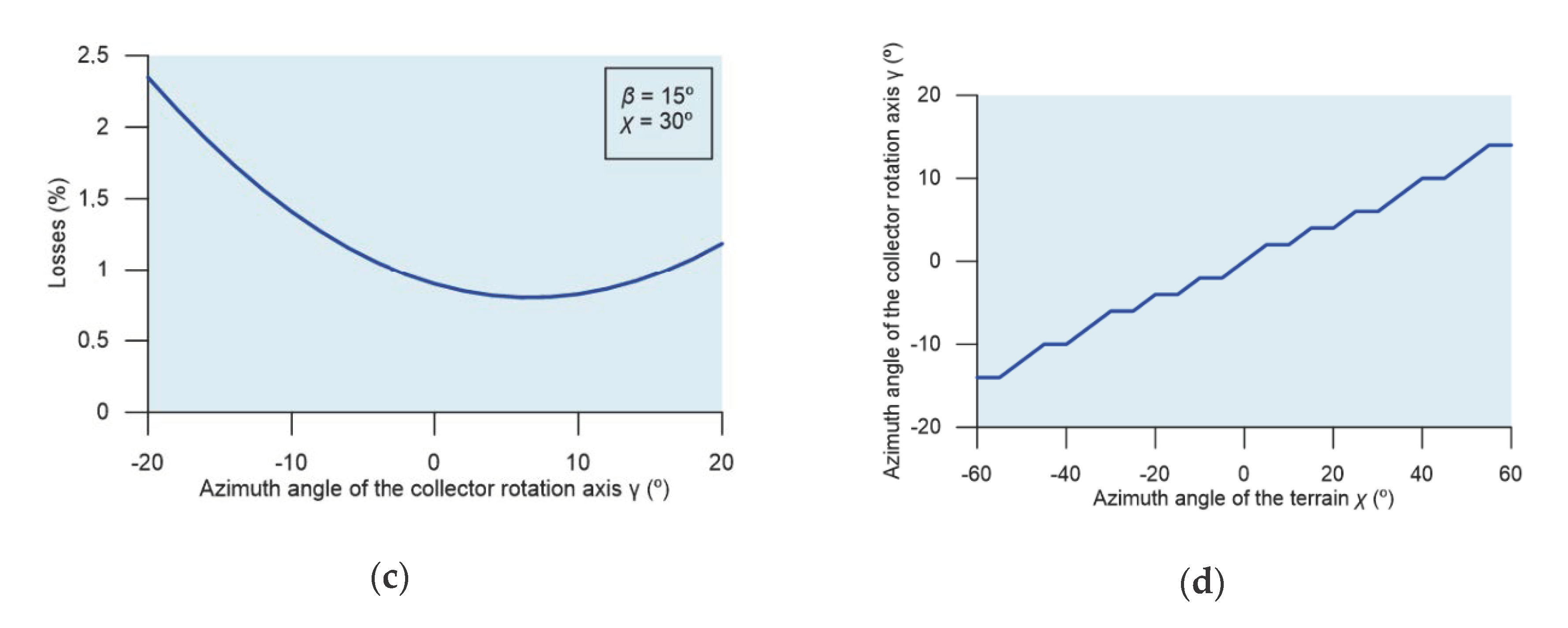Figure 9. Variation of the maximum radiation affecting the collector with respect to the inclination of the terrain.
Figure 9. Variation of the maximum radiation affecting the collector with respect to the inclination of the terrain.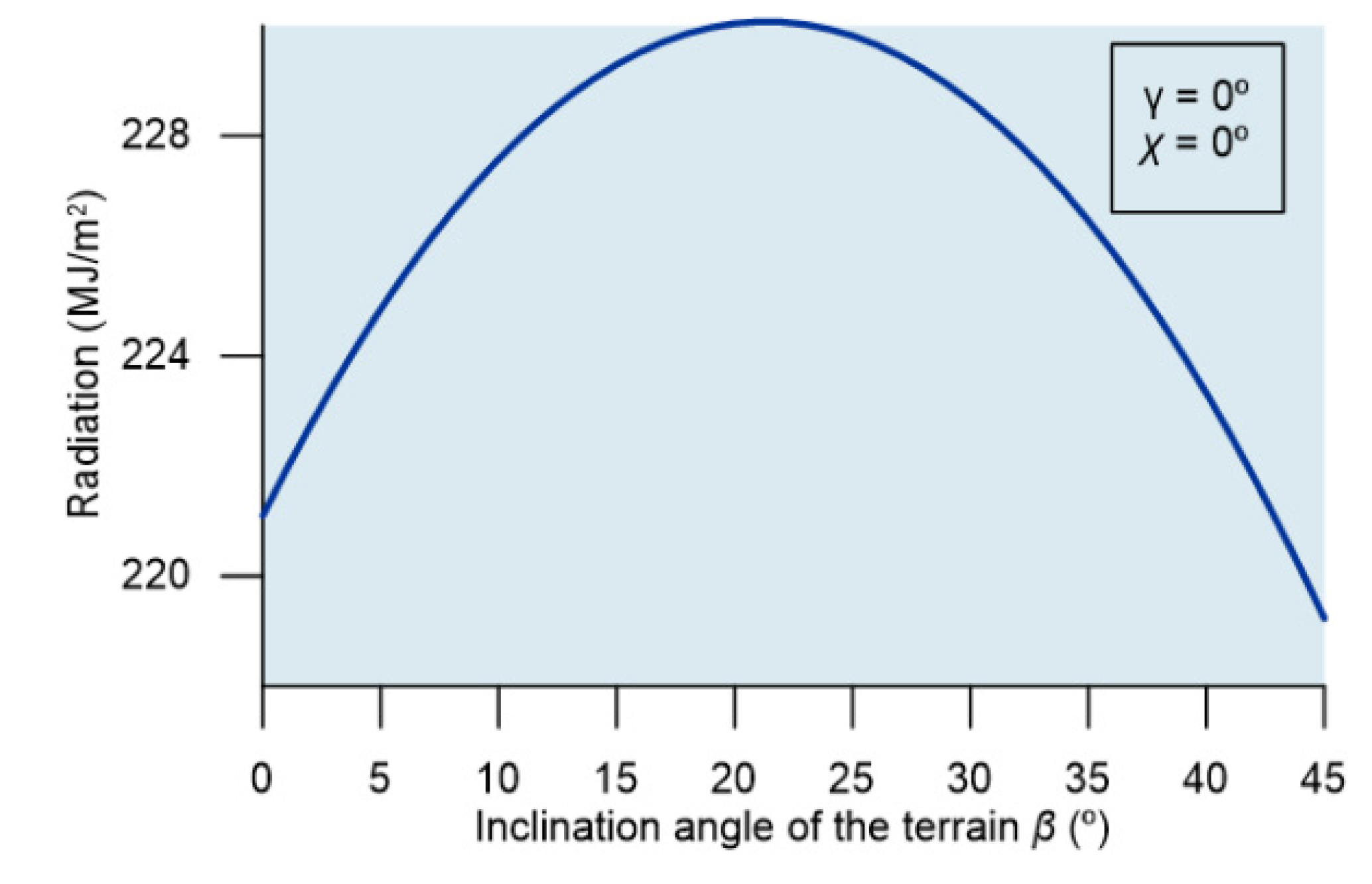Figure 10. Losses for different axis and terrain azimuth values.
Figure 10. Losses for different axis and terrain azimuth values.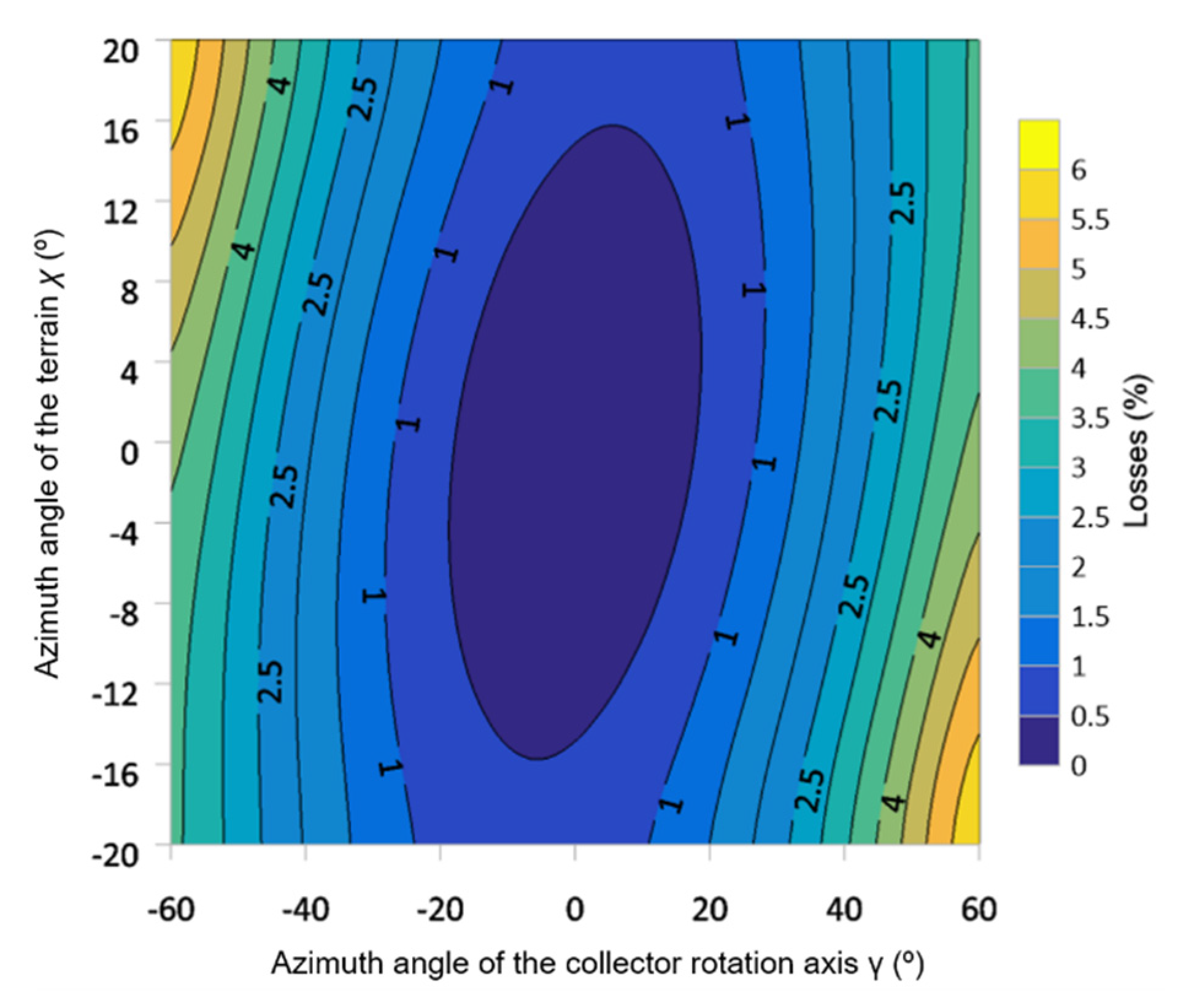Figure 11. Losses with 0° axis azimuth and 20° inclination.
Figure 11. Losses with 0° axis azimuth and 20° inclination.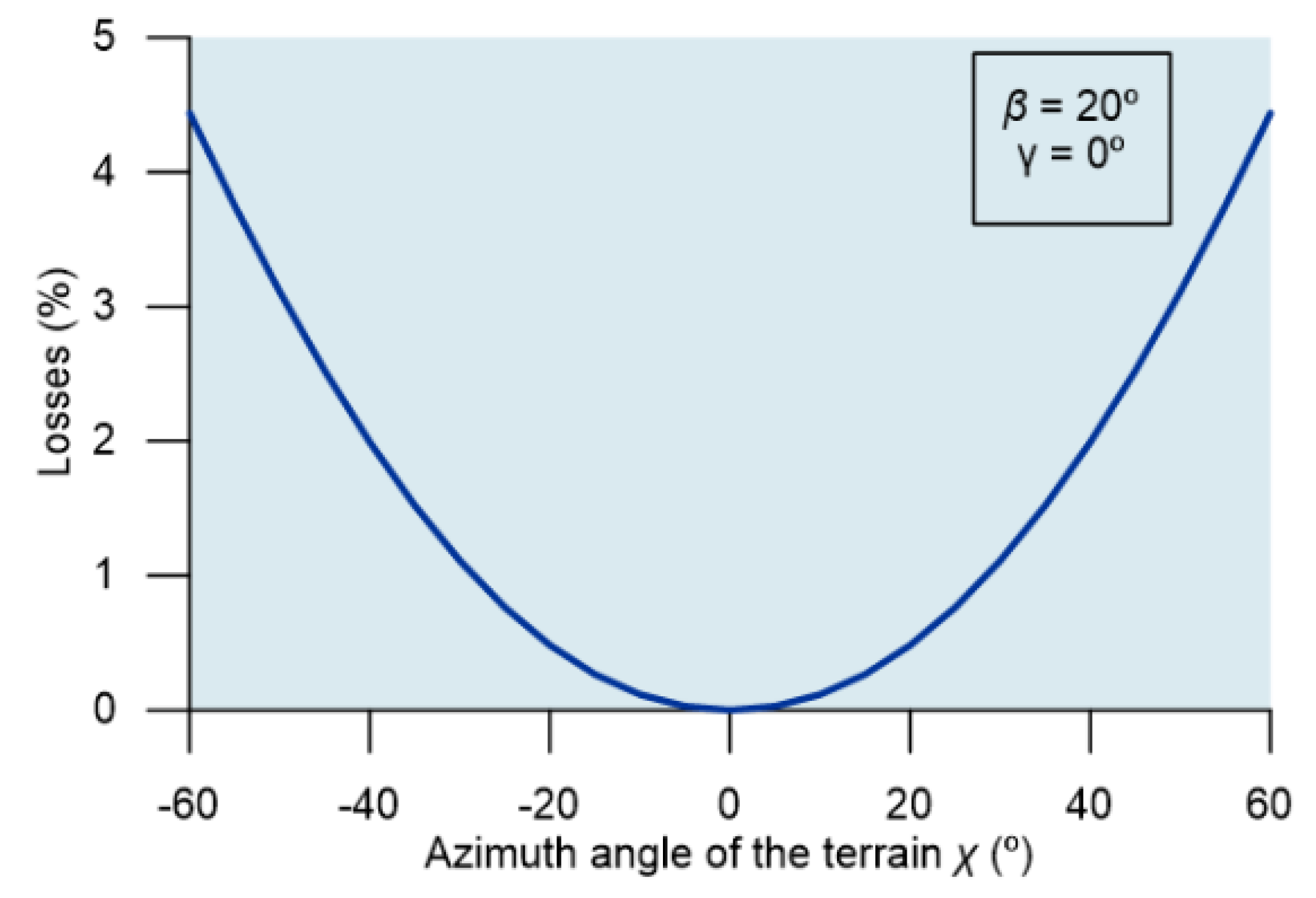Figure 12. Irradiance by varying the ratio d/h (h = 3 m).
Figure 12. Irradiance by varying the ratio d/h (h = 3 m).Publisher’s Note: MDPI stays neutral with regard to jurisdictional claims in published maps and institutional affiliations.